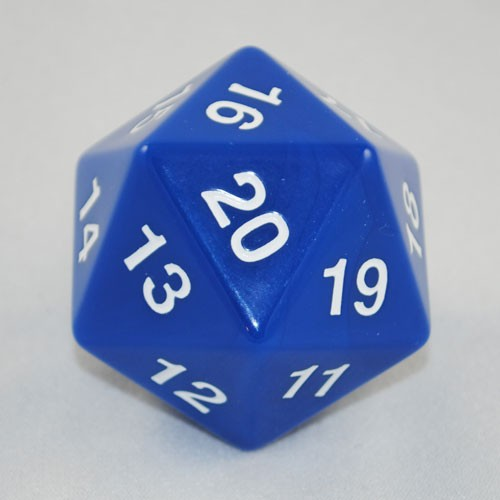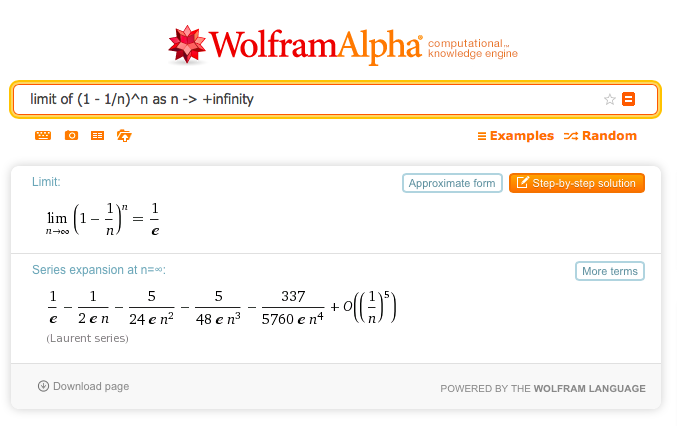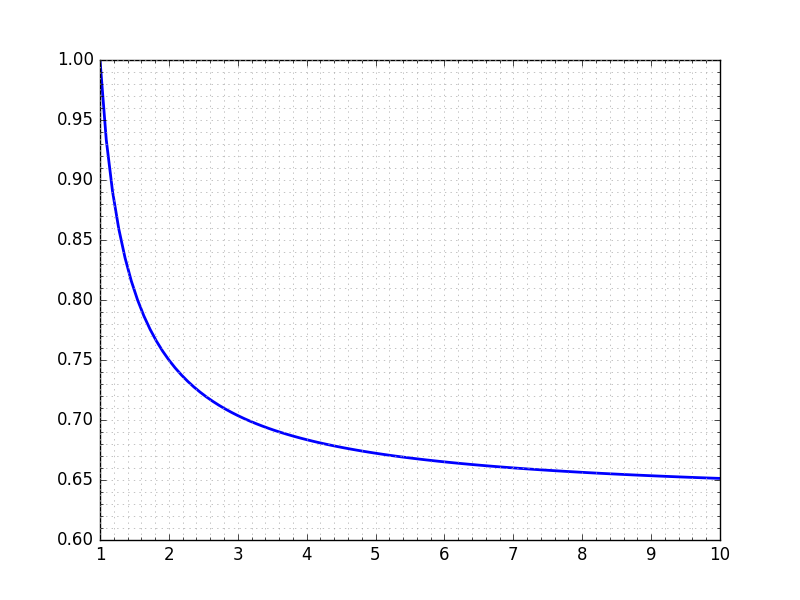# Rolls and runs

While writing my recent post on the hypergeometric distribution, I kept thinking about a particular approximation that I’ve run into fairly often. It didn’t really fit in that post, though, so I saved it for this one.

It comes up in the study of Bernoulli sequences, independent repeated trials in which there are two outcomes—yes/no, on/off, success/failure—and for which the probability of success, $$p$$, is the same for each trial. Bernoulli sequences lead a couple of practically important probability distributions:

• The binomial distribution, which covers the probability of $$m$$ successes in $$n$$ trials:

$P(m \mathrm{\ successes\ in\ } n \mathrm{\ trials}) = \binom{n}{m} p^m (1 - p)^{n-m}$

where $$\binom{n}{m}$$ is the binomial coefficient.

• The geometric distribution, which covers the probability of the first success occurring on the $$n^{th}$$ trial:

$P(\mathrm{first\ success\ in\ trial\ } n) = p (1 - p)^{n-1}$

You can see the similarities between the two distributions. The key differences are:

• The geometric distribution deals with a sequence with only one success, while the binomial handles sequences with any number of successes.
• In the sequence covered by the geometric distribution, the success occurs only on the $$n^{th}$$ trial, whereas the binomial covers sequences where success can occur in any of the $$n$$ trials.

Let’s consider a simple Bernoulli sequence familiar to board game enthusiasts: a series of rolls of a twenty-sided die (known to the cognoscenti as a D20).Image from Game Master Dice.

You might object to characterizing this as a Bernoulli sequence. After all, there are twenty possible outcomes from a roll of this die, not two. But let’s say we’re interesting in a particular outcome: lucky seven. Then we can consider each roll to have one of two outcomes, either seven (success) or not-seven (failure). With this definition, $$p = \frac{1}{20} = 0.05$$.

It doesn’t take a Bernoulli to figure out that a seven comes up, on the average, once every twenty rolls. But that tells us nothing about probabilities. For example, what if we want to know how many rolls are needed to have at least a 50–50 chance at getting a seven?

There are a couple of ways we can go about it, but first we have to define the problem a little more carefully. If our goal is roll a seven, and we were to actually run this experiment by rolling a D20 repeatedly, we’d stop as soon as we got a seven. So when I said “How many rolls are needed to have at least a 50–50 chance at getting a seven?” what I meant, in more precise terms, was “What is the minimum number of rolls needed to have at least a 50–50 chance at getting at least one seven?” That’s the question we’ll answer.

One way to go about it is the brute force method. Using the geometric distribution, we can figure out the probability that we get a seven on the first roll. That’s just $$0.05$$. Since that’s quite a bit less than our goal of $$0.50$$, we continue.

The probability that we first get a seven on the second roll is $$0.05 \times 0.95 = 0.0475$$. The probability that that we roll a seven on either the first or second rolls is the sum, $$0.0975$$. That’s still quite a way from our goal, so we continue. But let’s let the computer do it for us. Here’s a quick script:

python:
1:  #!/usr/bin/env python
2:
3:  p = .05
4:  sum = 0
5:  for n in range(1, 20):
6:    sum += p*(1 - p)**(n - 1)
7:    print "n = {:2d}:   {:5.3f}".format(n, sum)
8:    if sum > 0.50:
9:      break


The output is

n =  1:   0.050
n =  2:   0.098
n =  3:   0.143
n =  4:   0.185
n =  5:   0.226
n =  6:   0.265
n =  7:   0.302
n =  8:   0.337
n =  9:   0.370
n = 10:   0.401
n = 11:   0.431
n = 12:   0.460
n = 13:   0.487
n = 14:   0.512


So we can say we need at least 14 rolls to have at least a 50–50 chance of rolling at least one seven.

There’s a faster and more elegant way to solve this, though. Instead of thinking about rolling at least one seven, let’s think about the inverse problem. The probability of rolling no sevens in $$n$$ rolls is

$\binom{n}{0} p^0 (1 - p)^n = 0.95^n$

Therefore, the probability of rolling at least one seven in $$n$$ rolls is

$1 - 0.95^n$

and we can then solve

$1 - 0.95^n = 0.50$

for $$n$$ by using logarithms. That gives us

$n = \frac{\log 0.50}{\log 0.95} = 13.51$

Since we can’t roll our D20 13.51 times, that gives us 14 rolls, the same answer as before. Also

$1 - 0.95^{14} = 0.512$

which matches the probability we got through brute force.

If you’re wondering what kind of logarithms I used in the equation above, the answer is that it doesn’t matter. Natural logs, base 10 logs, base 2 logs—whatever you choose, the answer will be 13.51 because the ratio of the logs will be the same no matter what the base.1

Which leads us, at long last, to the approximation I wanted to talk about. We said before that the average number of rolls to get a seven is twenty. What is the probability that we’ll get at least one seven in twenty rolls? Based on what we’ve just done, it’s obviously more than 50%, but how much more?

If you walked up to me on the street and asked this question, I’d tell you without hesitation “about 63%.” If you had a 100-sided die and asked me the probability of getting at least one seven in 100 rolls, I’d give the same answer. For any problem where the probability is of the form

$1 - \left( 1 - \frac{1}{n} \right)^n$

my answer will be “about 63%” as long as $$n$$ is more than about 5 or so. Why?

Let’s first see how close my approximation is for the D20. The true answer is

$1 - 0.95^{20} = 0.6415$

Pretty close, eh?

63% is a close approximation because

$\lim_{n \to \infty} \left( 1 - \frac{1}{n} \right)^n = \frac{1}{e}$

and

$1 - \frac{1}{e} = 0.632$

You can check the limit yourself on Wolfram Alpha.Of course, the fact that the answer converges to 0.632 doesn’t tell us anything about how fast it converges. But it’s easy enough to see that by plotting the function.2As you can see, the convergence is pretty darned quick. Even for $$n = 3$$, the 63% approximation isn’t too far off.

Given that the convergence is from above, I suppose I could make my approximation more accurate by shifting it up a percentage point or two for small values of $$n$$.

1. Yes, the base has to be the same for both the numerator and the denominator.

2. Strictly speaking, I should be plotting only integer values along the abscissa, but because my quick-and-dirty plotting script uses a continuous domain (and I’m lazy) that’s what I’m presenting.